# Least-number operator

(diff) ← Older revision | Latest revision (diff) | Newer revision → (diff)-operator, minimization operator

A device for constructing new functions out of others, as follows. Letbe an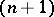-ary arithmetic function, i.e. a function with arguments and values in the set of natural numbers. It is assumed here thatis a partial function, i.e. it is not necessarily defined for all values of its arguments. One says that the-ary arithmetic functionis obtained fromby the least-number operator if, for any natural numbers,if and only if the values of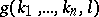are defined and are not equal to zero for all, whileis defined and equals zero. Ifis obtained fromby the least-number operator, one writes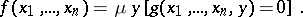An important property of the least-number operator is the following: Ifis a computable function, then the above functionis always partially computable. In fact, if there is an algorithm computing, then the value ofcan be computed as follows. Compute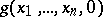. If the computation process ends, i.e. ifis defined, and if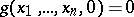, put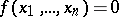; but if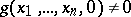, begin to compute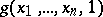. If the process ends and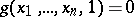, put; but if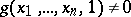, proceed to compute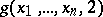; etc. The computation will come to an end if there exists asuch that, for all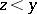,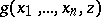is defined and not zero, while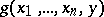is defined and equal to zero. Then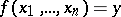.

The least-number operator plays an important role in the definition of the class of partial recursive functions (cf. Partial recursive function).